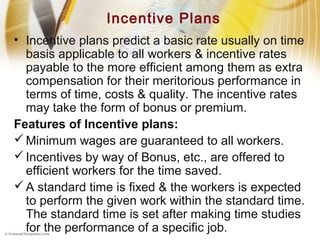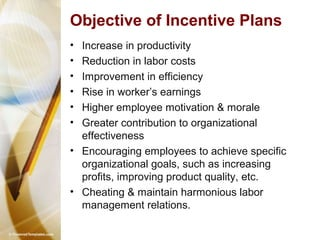Successfully reported this slideshow.

# Incentives

28

Share×
1 of 20
1 of 20

# Incentives

28

Share

## More Related Content

### Related Books

Free with a 14 day trial from Scribd

See all

### Related Audiobooks

Free with a 14 day trial from Scribd

See all

### Incentives

1. 1. INCENTIVE PAYMENTS
2. 2. Incentive Plans • Incentive plans predict a basic rate usually on time basis applicable to all workers & incentive rates payable to the more efficient among them as extra compensation for their meritorious performance in terms of time, costs & quality. The incentive rates may take the form of bonus or premium. Features of Incentive plans: Minimum wages are guaranteed to all workers. Incentives by way of Bonus, etc., are offered to efficient workers for the time saved. A standard time is fixed & the workers is expected to perform the given work within the standard time. The standard time is set after making time studies for the performance of a specific job.
3. 3. Objective of Incentive Plans • Increase in productivity • Reduction in labor costs • Improvement in efficiency • Rise in worker’s earnings • Higher employee motivation & morale • Greater contribution to organizational effectiveness • Encouraging employees to achieve specific organizational goals, such as increasing profits, improving product quality, etc. • Cheating & maintain harmonious labor management relations.
4. 4. Prerequisites for An Effective Incentive System • The Co-operation of workers in the implementation of an incentive scheme. • The scheme must be based on scientific work measurement. • Indirect workers, such as supervisors, foremen, charge hands, helpers, crane operators, canteen staff, store keepers & clerical staff should also be covered by incentive schemes. • There should be management commitment to the cost & time necessary to administer incentive scheme. • Greater need for planning incentive scheme. Cont…..
5. 5. Other Safeguards are: • Appropriate to the type of work carried out & workers employed. • The reward should be clearly & closely linked to the efforts of the individual of group. • Individual or groups should be able to calculate the reward they get at each of the levels of output they are capable of achieving. • Individuals or groups should have a reasonable amount of control over their efforts & therefore their rewards. • The scheme should operate by means of well-defined & easily understood formula. • The scheme should properly installed & maintained. • Provisions should be made for controlling the amounts paid, to ensure that they are proportionate to effort. • Provisions should be made for amending rates in defined circumstances. • Create incentives for performance & disincentives for non- performance. • Set & review specific objectives for each employee periodically.
6. 6. Scope of Incentive Schemes • INCENTIVE SHEMES ARE DIFFICULT TO APPLY IN: Industries in which measurement of individual or group output is rendered difficult or impossible either by technical consideration or by psychological circumstances which might be prejudicial to output; Industries in which the control of quality is necessary & is particularly difficult, or in the case of certain classes of workers, where high quality & accuracy of work is of prime importance & Industries in which the work is dangerous & it is particularly difficult to ensue the observance of adequate safety precautions
7. 7. Types of Incentive Schemes • The International Labor Organization (ILO) classifies it in four types: Income vary in Proportion to Output Income vary less Proportionately than Output Income vary Proportionately more than Output Income differ at different levels of Output.
8. 8. Types of Incentive Schemes Incentive Schemes Earnings vary in the same proportion as output Earnings vary less Proportionately than output Earnings vary Proportionately more than output Earnings differ At different Levels of output Straight Piece Work Standard Hour Halsey Plan Rowan plan High Piece Rate High Standard Hour Taylor's Differential Piece Rate Merrick Differential Piece Rate Barth Scheme Bedaux Plan Gantt Task System Emerson’s Efficiency plan
9. 9. Straight Piece Work: It is a simplest & oldest method. Here, Rate per unit of output is fixed & the total earnings of worker are arrived at by multiplying the total output by the rate per unit. E.g.: If the rate per unit is 10 paisa & the total output of an employee is 100 units, his or her earnings will be 100*0.10=Rs. 10.00 Standard Hour: Also called “100 percent gains-sharing.” Standard time in terms of hours is fixed for completion of a job. The rate per hour is then determined. E.g. Standard Time: 10hrs Rate per hour= Rs. 1 Case (i) Time taken = 8hrs Earnings = 10*1=Rs 10.00 Case (ii) Time taken = 12 hrs Earnings = 12*1=Rs 12.00 (If time wages are guaranteed)
10. 10. • Halsey Plan : Recognizes individual efficiency & pays bonus on the basis of time saved. Main features are: Standard time is fixed for each job or operation. Time rate is guaranteed & the worker receives the guaranteed wages irrespective of whether he completes the work in the time allowed or takes more time to do the same. If the job is completed in less than the standard time, the worker is paid a bonus of 50% of time saved at time rate in addition to his normal time wages. Total Earnings = Time Taken* Hourly Rate + Bonus Bonus = 50% of time saved (1/2*Time Saved*Hourly Rate)
11. 11. • Rowan Plan: Bonus paid to the employee is equal to the proportion of the time saved to the standard time. Time Saved Total Earnings = Time Taken * Hourly Rate + Bonus E.g. Std Time =10hrs Rate per Hr = Rs1 Time Taken = 8hrs Bonus = 2/10*8*1 =Rs 1.60 Earnings = 8*1+1.60 =Rs. 8 =Rs 9.60 Bonus = Std. Time Time Taken Hourly Rate
12. 12. • Barth Scheme : does not guarantee the time – rate. The worker’s pay is determined by multiplying the standard hour by the number of hours actually taken to do the job, taking the square root of the product & multiplying it by the worker’s hourly rate. Wages = Standards Time* Time Taken *Hourly Rate • E.g. Std time = 10hrs Rate per hour = Rs.1 Time Taken = 8 hrs Earnings = 8*10 = 8.94 * 1 = Rs 8.94
13. 13. • Bedaux Scheme: Standard time for the job is fixed. Each minute of the standard time is called a point or B. Each job has a standard number of Bs. The worker receives bonus which is equal to 75% of the number of points earned, in excess of 60 per hour, multiplied by one sixtieth of the worker’s hourly rate. E.g. Std Time = 10 hrs Rate per Hour = Rs.1 Actual time = 8 hrs Earnings : Time Wage = 8*1 = Rs.8.00 Bonus: Std Bs = 10*60 = 600 Actual Bs = 8*60 = 480 Bs Saved = 120 Bonus 75 120 1 100 60 = Rs. 1.50 Total Earnings = 8+ 1.50 = Rs. 9.50
14. 14. • Earnings Varying Proportionately More than Output: This category includes two methods (i) High Piece Rate (ii) High Standard Hour System
15. 15. Earnings differing at Different Levels of Outputs: • Taylor’s Differential Piece Rate System: There is low rate for output below the standard, and a higher piece-rate for output above the standard with a large bonus of 50% of the time-rate when the standard output is attained. To illustrate : Standard Output = 100 units Rate per Unit = 10 paise Differentials to be applied: 120 percent of piece-rate at or above the standard 80 percent of piece-rate when below the standard Case (i) Output = 120 units Earnings = 120*(120/100)*0.10= Rs.14.40 Case (ii) Output = 90 units Earnings = 90*(80/100)*0.10=7.20
16. 16. • Merrick Differential Piece–Rate System: It is a modification of earlier system with three instead of two rates. E.g. Std Output = 100 units Piece Rate = 10 paise Case (i) Output= 80units Efficiency = 80/100*100=80% Earnings: As the efficiency is less than 83%, only base piece-rate applies: 80*.10=8.00 Case (ii) Output = 90 units Efficiency = 90/100*100=90% Earnings: As the efficiency is more than 83% but less than 100%, 110% of the base piece-rate applies: 90*(110/100)*0.10=9.90 Case (ii) Output = 110 units Efficiency = 110/100*100=110% Earnings: As the efficiency exceeds 100%, 120% of the base piece- rate applies: 90*(120/100)*0.10=13.20
17. 17. • Gantt Task System: The worker is guaranteed his /her time-rate for output below the standard. On reaching the std output or task, which is set at a high level, the worker is entitled to a bonus of 20% of time wages. It operates as follows: Rate per hr = Rs 0.50 High piece-rate = Rs 0.10 Std Output = 80 units Time Taken = 8hrs Case (i) Output = 70 units As the output is less than the standard only time wages are paid to the worker. Earnings = 8*.50= Rs 4.00 Case (ii) Output = 80 Units Earnings: As output is equal to the standard, the worker is entitled to time wages plus 20% of time wages as bonus. Time wages = 8*.50=4.00 Bonus = 20/100*4=Rs 0.80 Total Earnings = Rs.4.80 Case (iii) Output earnings= 110 Units As the output is more than the standard, the worker is entitled to a high piece- rate. 110*.10= Rs.11.00
18. 18. • Emerson’s Plan: A standard is set for the job, & efficiency of each worker is determined by dividing the time taken by the std time. • Illustration: Std Output in 10 hrs = 100 units Rate per hr = Rs 1 Case (i) Output in 10 hrs = 50 units Earnings: Efficiency = 50% As efficiency is below 67 % the worker is entitled to time wages only. 10*1=Rs 10.00 Case (ii) Output in 10 hrs = 100 units Earnings: Efficiency = 100% The worker is entitled to time wages plus 20% of time wages as bonus. Time Wages = 10*1 =Rs 10.00 Bonus = 20/100*10 =Rs 2.00 Earnings: = 10 + 2 =Rs12.00 Case (iii) Output in 10 hrs = 130 units Earnings: Efficiency = 130% At the rate of 20% at 100% efficiency & one percent increase for every one percent increase in efficiency, the worker is eligible for 50% of the time wage as bonus. Time Wages = 10*1 =Rs 10.00 Bonus = 50/100*10 =Rs 5.00 Earnings: = 10 + 5 =Rs15.00
19. 19. Advantages:  Better co-operation among workers  Less Supervision  Reduced incidence of absenteeism  Reduced clerical work &  Shorter training time. Disadvantages:  An efficient worker may be penalized for the inefficiency of the other members.  The incentive may not be strong enough to serve its purpose &  Rivalry among the members of the group defeats the very purpose of team work & co-operation. Group Incentive
20. 20. Incentive Schemes in Indian Industries • Introduces in 1946 • Not identical within industries also. • Modifications done as per the suitability. • Still in infancy stages